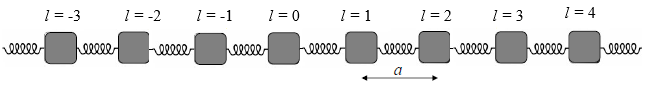Menu Outline Exercise Questions Appendices Lectures Student Projects Books Sections Introduction Atoms Molecules Crystal Structure Crystal Diffraction Crystal Binding Photons Phonons Electrons Band Model Crystal Physics Semiconductors

PHY.K02UF Molecular and Solid State Physics

## 1-d chain of atoms

The simplest crystal that can be used to calculate phonon properties is a chain of equally spaced atoms confined to move in one dimension.For small displacements of the atoms from their equilibrium position, the bond potentials can be approximated by linear springs. Newton's law for this system is,

$\begin{equation} M\frac{d^2u_l}{dt^2}=C(u_{l-1}-u_l)+C(u_{l+1}-u_l). \end{equation}$

Here $M$ is the mass of the atoms, $u_l$ is the displacement of atom $l$ from its equilibrium position, and $C$ is the spring constant. To find the normal modes, we assume that all of the atoms move with the same frequency $u_l=A_l\exp(i\omega t)$. Here $A_l$ is the amplitude of the motion of atom $l$ and $\omega$ is the angular freqeuncy. These equations are written out in matrix form for six atoms with period boundary conditions,

\begin{equation} \left[ \begin{matrix} 2C-\omega^2M & -C & 0 & 0 & 0 & -C \\ -C & 2C-\omega^2M & -C & 0 & 0 & 0 \\ 0 & -C & 2C-\omega^2M & -C & 0 & 0 \\ 0 & 0 & -C & 2C-\omega^2M & -C & 0 \\ 0 & 0 & 0 & -C & 2C-\omega^2M & -C \\ -C & 0 & 0 & 0 & -C & 2C-\omega^2M \\ \end{matrix} \right]\left[ \begin{matrix} A_1 \\ A_2 \\ A_3 \\ A_4 \\ A_5 \\ A_6 \end{matrix} \right]=0. \end{equation}

This matrix equation can be written in terms of the translation operator $\textbf{T}$ and the identity matrix $\textbf{I}$,

$\begin{equation} \left[(2C-\omega^2M)\textbf{I}-C(\textbf{T}+\textbf{T}^{-1})\right]\vec{A}=0 \end{equation}$

Thus the eigenvectors that we seek are also eigenvectors of the translation operator. The eigenvectors of the translation operator are,

\begin{equation} \left[ \begin{matrix} 0 & 1 & 0 & 0 & 0 & 0 \\ 0 & 0 & 1 & 0 & 0 & 0 \\ 0 & 0 & 0 & 1 & 0 & 0 \\ 0 & 0 & 0 & 0 & 1 & 0 \\ 0 & 0 & 0 & 0 & 0 & 1 \\ 1 & 0 & 0 & 0 & 0 & 0 \end{matrix} \right]\left[ \begin{matrix} 1 \\ e^{ika}\\ e^{i2ka} \\ e^{i3ka} \\ e^{-i2ka} \\ e^{-ika} \end{matrix} \right]=\left[ \begin{matrix} e^{ika}\\ e^{i2ka} \\ e^{i3ka} \\ e^{-i2ka} \\ e^{-ika} \\ 1 \end{matrix} \right] = e^{ika}\left[ \begin{matrix} 1 \\ e^{ika}\\ e^{i2ka} \\ e^{i3ka} \\ e^{-i2ka} \\ e^{-ika} \end{matrix} \right]\hspace{1.5cm}k=-\frac{4\pi}{L},-\frac{2\pi}{L},0,\frac{2\pi}{L},\frac{4\pi}{L},\frac{6\pi}{L}. \end{equation}

Each eigenvector of the translation operator is labeled by a wavenumber $k$. The normal modes that we seek have the form,

$\begin{equation} u_l=A_k\exp\left(i(lka-\omega t)\right). \end{equation}$

Substituting this solution into the differential equation yields,

$\begin{equation} -M\omega^2A_k\exp\left(i(lka-\omega t)\right)=C(A_k\exp\left(i((l-1)ka-\omega t)\right)-A_k\exp\left(i(lka-\omega t)\right))+C(A_k\exp\left(i((l+1)ka-\omega t)\right)-A_k\exp\left(i(lka-\omega t)\right)). \end{equation}$

A factor of $A_k\exp\left(i(lka-\omega t)\right)$ can be cancelled out,

$\begin{equation} -M\omega^2=C(\exp(-ika)+\exp(ika)-2). \end{equation}$

The exponential functions can be written as a cosine $\exp(ix)+\exp(-ix) = 2\cos(x)$,

$\begin{equation} M\omega^2=2C(1-\cos(ka)). \end{equation}$

Using the half-angle formula $\sin ^2\left(\frac{x}{2}\right) = \frac{1}{2}(1-\cos x)$ this be written,

$\begin{equation} \omega=\sqrt{\frac{4C}{M}}\sin\left(\frac{|ka|}{2}\right). \end{equation}$
 $\large \frac{\omega}{\sqrt{\frac{4C}{m}}}$ $ka$

Your browser does not support the canvas element.
$ka=$

The and buttons let you change the wavenumber $k$ to display different normal modes.

In the normal mode specified by $k$, the motion of atom $l$ is proportional to,

$\begin{equation} \cos (-\omega t +lka). \end{equation}$

The normal modes have wave-like solutions with a speed $c=\omega /|\vec{k}|$. If $\omega \propto |\vec{k}|$ then all of the waves move at the same speed and any wave pulse constructed by adding the normal modes together will keep its shape. For the case of a linear chain of atoms the different normal modes travel at different speeds so that if a wave pulse is constructed from these normal modes, the different components of the pulse will travel at different speeds and the pulse will get wider with time. For this reason the relationship between $\omega$ and $\vec{k}$ is called the dispersion relation as it describes how a pulse will disperse (spread out).

The steps we take to determine the phonon dispersion relation are:

• Write down the equations of motion (Newton's law) for a system of masses connected by linear springs.
• Note that because of translational symmetry, the normal modes are eigenfunctions of the translation operator. The normal modes are labeled by their wave vector $\vec{k}$.
• Plug the normal mode solutions into the equations of motion. This results in the dispersion relation $\omega(\vec{k})$.